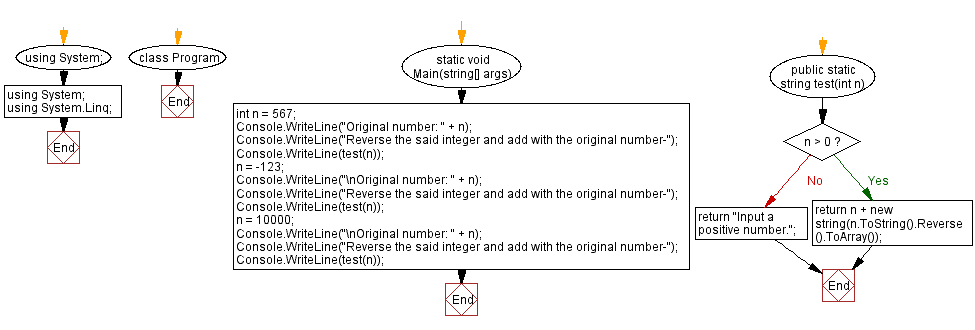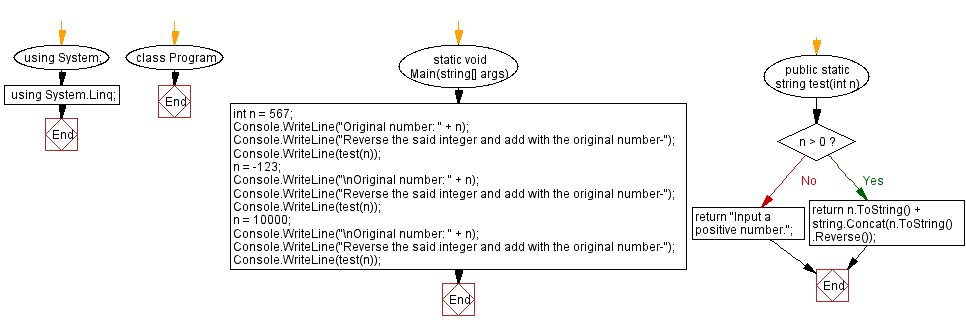﻿ C# - Reverse an integer and add with the original number# C# Sharp Exercises: Reverse an integer and add with the original number

## C# Sharp String: Exercise-59 with Solution

Write a C# Sharp program to reverse an positive integer and add the reverse number with the original number.

Sample Data:
(456) - > 456654
(-123) -> "Input a positive number."
(10000) -> 1000000001

Sample Solution-1:

C# Sharp Code:

``````using System;
using System.Linq;
namespace exercises
{
class Program
{
static void Main(string[] args)
{

int n = 567;
Console.WriteLine("Original number: " + n);
Console.WriteLine("Reverse the said integer and add with the original number-");
Console.WriteLine(test(n));
n = -123;
Console.WriteLine("\nOriginal number: " + n);
Console.WriteLine("Reverse the said integer and add with the original number-");
Console.WriteLine(test(n));
n = 10000;
Console.WriteLine("\nOriginal number: " + n);
Console.WriteLine("Reverse the said integer and add with the original number-");
Console.WriteLine(test(n));
}

public static string test(int n)

{
if (n > 0)
{
return n + new string(n.ToString().Reverse().ToArray());
}
return "Input a positive number.";
}
}
}
```
```

Sample Output:

```Original number: 567
Reverse the said integer and add with the original number-
567765

Original number: -123
Reverse the said integer and add with the original number-
Input a positive number.

Original number: 10000
Reverse the said integer and add with the original number-
1000000001

```

Flowchart :Sample Solution-2:

C# Sharp Code:

``````using System;
using System.Linq;
namespace exercises
{
class Program
{
static void Main(string[] args)
{

int n = 567;
Console.WriteLine("Original number: " + n);
Console.WriteLine("Reverse the said integer and add with the original number-");
Console.WriteLine(test(n));
n = -123;
Console.WriteLine("\nOriginal number: " + n);
Console.WriteLine("Reverse the said integer and add with the original number-");
Console.WriteLine(test(n));
n = 10000;
Console.WriteLine("\nOriginal number: " + n);
Console.WriteLine("Reverse the said integer and add with the original number-");
Console.WriteLine(test(n));
}

public static string test(int n)

{
if (n > 0)
{
return n.ToString() + string.Concat(n.ToString().Reverse());
}
return "Input a positive number.";
}
}
}
```
```

Sample Output:

```Original number: 567
Reverse the said integer and add with the original number-
567765

Original number: -123
Reverse the said integer and add with the original number-
Input a positive number.

Original number: 10000
Reverse the said integer and add with the original number-
1000000001
```

Flowchart :C# Sharp Code Editor:

Improve this sample solution and post your code through Disqus

What is the difficulty level of this exercise?

Test your Programming skills with w3resource's quiz.

﻿